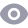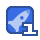### 51单片机串口通信程序 含详细例子22收藏

TL= unsigned char)(256-(XTAL/(③32L*12L* baudrate));∥定时器0赋初值 SCON 0x50 PCON =0X00 TR1=1 旧=0x00;∥禁止任何中断 while(1) Whe(R|=0); R|=0: C=SBUF;∥从缓冲区中把接收的字符放入c中 SBUF=c;∥要发送的字符放入缓冲区 while(tI== 0) T=0; 4mmrm17 / E51Proc asy51Pro编程器主程序,负责通讯,管理编程操作 #include <e51 Pro. h> BYTE ComBuf18];∥/串口通讯数据缓存,发送和接收都使用 U| NT aDdress;/ROM中地址计数 UINT tImeout;超时计数 ProWork pw;/编程器一般撅作 void delay_ us( BYTE nUS川微秒纵延时<255us TH0=0; TL0=0 TR0=1; While(TL0<ηUs)/利用T0做定时计数器,循环采样,直到达到定时值 TR0=0; void Delay_msU|TnMs川豪秒级的延时<65535ms UINT n=0 TR0=1; while(n<nMs)∥/利用T0做定吋计数器,循环采样,直到达到定时值 TH0=0 while( THo<4) TRO= BooL Waitcomm等待上位机的命令,18字节 BYTEn=0 R|=0: while(!R)y∥等待第一个字节 ComBuf[n]=SBUF; R|=0 n++; for(n;n≤=17n++) tImeoUt while(!R tImeout++ f( tImeoUt>10000后17个字节都有超时限制 return 0 ComBuf[n=SBUF R|=0: return 1 BOOL WaitResp(等待上位机回应,1字节,有超时限制 nTime out=0 R|=0 while (!Ri) nTime○ut++; if(n T ime Out>50000) return o R|=0 ComBuf[O=SBUF return 1 BOoL WaitData()∥写器件时等待上位机数据,18字节,有超时限制 BYTE: R|=0 for(n=0; n<=17: n++) nTime○ut=0 while(!rl) nTime out++. if(n TimeOut> 10000) return o R|= ComBufl buF return 1 void senddata(y发送数据或回应操作完成,18字节 BYTE n=o for(n;n≤=17n++) T|=0 SBUF=Com Buf while(!TDy Tl=0; void Send Resp(/应上位机1个字节,在写器件函数中使用 T=0 SBUF=Com Buf[O] while(!Tit T=0 void SetVpp5VO∥/设置Vpp为5v P34=0; void SetVppOV()设置Vpp为0v P33=0 P34=1; void SetVpp12V(设置pp为12v P33=1; void RstProo/编程器复位 pw. fpProOver)∥/直接编程结束 Send Data();/通知上位机,表示编程器就绪,可以直接用此函数因为协议号( Com Buf[O门)还没被修改,下同 void Readsign()/读特征宇 pw. fpReadsign(; Send data();/通知上位机,送出读出器件特征字 void erase(川∥擦除器件 pw.fpEraseo Send data();/通知上位机,擦除了器件 void write(∥写器件 BYTE: pw. fplnitPro()/编程前的准备工作 Send data(;/应上位机表示进入写器件状态, 可以发米数据 while(1) f(WaitData(/如果等待数据成功 f( ComBuf0}=0X07川∥判断是否继续写 for(n=2;n<=17;n++) Com Buf2~17为待写入数据块 if( pw fpWrite(com Buf[n])∥<调用写该器件一个单元的函数 pw. fpProovert();/出错了就结束编程 Com Buf[O]=oXff SendResp(O,回应上位机个字节, 表示写数据出错了 WaitData();/等待上位机的回应后就结束 return aDdress++;//下一个单元 ComBuf[O]=1;/回应上位机一个字节,表示数据块顺利亢成,请求继续 SendRespo else if( ComBufl]==000)∥写器件结束 break else/能是通讯出错了 pw. fpProover( return; else∥等待数据失败 pw. fpProOver(: return pw. fpProover(;/编程结束后的工作 Delay_ms(50);∥/延时等待上位机写线程结束 ComBuf]=0;/通知上位机编程器进入就绪状态 Send DataO void read()∥读器件 BYTE n pw.tplnitPro();元设置成编程状态 Send Data(;/应上位机表示进入读状态 while(1) f(WaitResp()∥/等待上位机回应1个字节 f( ComBuf[O]==0/ Com Bu]=0表示读结束 break else if(( ComBufl]=0xf)/)xf表小重发 aDdress=n Address-0x0010 for(n=2n<=17;n++∥ ComBuf[2-17保存读出的数据块 ComBuf[n= pw. fpRead();/<<≤≤≤调用写该器件一个单元的函数 aDdress++;/一个单元 ComBuf0]=6:/l上位机发送读出的数据块 Send Data( break;/待回应失败 pw.fpProover();操作结束设置为运行状态 Com Buf[o]=0;〃道知上位机编程器进入就绪状态 Send Data(; void lock()∥写锁定位 pw flOck Send Datao ∥支持的F|D,请在这里继续添加 extern void PrepareProl00):FD=00:AT89C51编程器 extern void Prepare Pro01);/FD=01AT89c2051编程器 extern void PreparePro02();∥FD=02AT89S51编程器 void main() SP=0×60 SetVpp5V();/先初始化Vpp为5v SCON=OXOO TCON=0x00 ∥PCON=0x00;∥波特率*2 E=0x00 //TMOD: GATEC/TM1MOGATEC/TM1MO 0100001 TMOD=0x21;/T0用于延时程序 TH1=0xf TL1=0x,/)特率28800*2,注意PCON //SCON: SMO SM1 SM2 RENITB8 RB8 TIRI 01010000 SCON=0X50 TR1=1; Delay_ms(1000)/延时1秒后编程器自举 ComBuf[O=0 Send Data0; Whie(1y/口通讯采用查询方式 f(! Waitcomm()∥/如果超时通讯出错 Delay ms(500) ComBuf(O-=0;/让编程器复位使编程器就绪 switch( ComBuf1y根据F|D设置( ProWork)pW中的函数指针 case 0: ∥lt89c51编程器 Prepare Pro00(; break case 1 ∥lt89c2051编程器 Prepare Pro010; break case 2. ∥lt89s51编程器 PreparePro02 0; break; ∥case3:支持新器件吋,请继续向下添加 l break ∥/case4: ∥/ break; default: ComBuf[O]=oxff Com Buf=0xf;∥示无效的操作 break switch( ComBuf]川根据操作|D跳到不同的操作函数 case 0x00 RstProt; break;/(程器复位 case oxo Reassign(; break;∥读特祉字 case x02 Erase(; break;∥擦瘵除器件 case 0x03 Wrte(; break;/写器件 case 0x04 Read) break;/读器件 case 0x05 Lock(); break;∥/写锁定位 default: Send Data(; break; void Init serial(void) TMOD=0x20;∥T1方式2 PCoN=0x00;∥PCON=00H,SMOD=0PD=PCON2=1进入扣电模式 TH1=1≡BAUD9600;∥BAUD:9600 SCON=0x50;∥串行通信方式1REN=1允许接收 ET1=0:∥/不允许中断 TR1=1;∥开启定时器1 ∥关闭所有中断允许位 mems(& serial Buf,0x00, SERIAL BUF LEN);∥/初始化 SerialBuf [ SERIAL BUF LEn] ★大kk大kk★大大木;;;大大kkk★古大*大大古k大大古kk;大大kk大k 名称: SendByte( 林*功能:串口发送一个字节 输入: cdAta *返回:无 说明:无 大★k古大内大大★★大大水古古大大古水大大★大古者大大大大大★木大大★内言大大古 void Send Byte(unsigned char ucData) SBUF cdAta while(!TI) CLRWDT, 6RS232串口通信程序 include sat89X52.H> unsigned char code dispcode1[=t welcome!3 unsignedcharcodedispcode2i=fwww.zhaoiian.comy unsigned char i,j, k, I, DData sbit rs= P3 5: sbit rw= p3 6 sbit e= p37: void delay {for(1=0;1<=100:1++){} void enable(//write order RS=O; RW=0; E=0; delay(; E=1; void enable()//write data RS=1; RW=0; E=0; dclay (; E= void initializtion //cd initializtion for(i=0;i<=100;i+ P0=OxO cnablcl P0=0x38 enable() PO=OxOf; enabled PO=0X06; enable

...展开详情

• 10
资源
• 14
粉丝
•等级

最新推荐51单片机串口通信程序 含详细例子 42积分/C币 立即下载
1/20试读结束， 可继续读2页

42积分/C币 立即下载 ＞